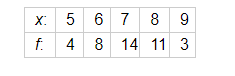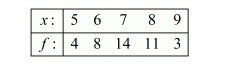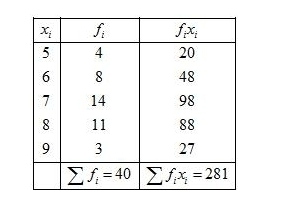# Calculate the mean for the following distribution :

Question:

Calculate the mean for the following distribution :Solution:

Given:First of all prepare the frequency table in such a way that its first column consist of the values of the variate $\left(x_{i}\right)$ and the second column the corresponding frequencies $\left(f_{i}\right)$.

Thereafter multiply the frequency of each row with corresponding values of variable to obtain third column containing $\left(f_{i} x_{i}\right)$.

Then, sum of all entries in the column second and denoted by $\sum f_{i}$ and in the third column to obtain $\sum f_{i} x_{i}$.We know that mean, $\bar{X}=\frac{\sum f_{i} x_{i}}{\sum f_{i}}$

$=\frac{281}{40}$

$=7.025$

Hence, mean $=7.025$